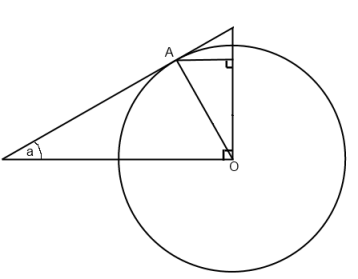#### You may also like### Degree Ceremony

Can you find the sum of the squared sine values?### Logosquares

Ten squares form regular rings either with adjacent or opposite vertices touching. Calculate the inner and outer radii of the rings that surround the squares.### Ball Bearings

If a is the radius of the axle, b the radius of each ball-bearing, and c the radius of the hub, why does the number of ball bearings n determine the ratio c/a? Find a formula for c/a in terms of n.

# Geometric Trig

##### Age 16 to 18 ShortChallenge LevelIn this diagram OA is a radius of a unit circle. The hypotenuse of the large triangle is tangent to the circle at A.

Find the lengths $\cos(a)$, $\sin(a)$, $\tan(a)$, $\frac{1}{\cos(a)}$, $\frac{1}{\sin(a)}$  and $\frac{1}{\tan(a)}$ in the diagram.

Find the areas of all of the regions in the diagram.

Did you know ... ?

Whilst trigonometric functions are defined algebraically in more advanced applications, geometric images such as this one can give great insight into the relationships between the functions. They also impart a sense of the beauty and interconnectedness of mathematics, which inspires many students of mathematics.